# KSEEB Solutions for Class 9 Science Chapter 4 Structure of The Atom

Students can Download Science Chapter 4 Structure of The Atom Questions and Answers, Notes Pdf, KSEEB Solutions for Class 9 Science helps you to revise the complete Karnataka State Board Syllabus and to clear all their doubts, score well in final exams.

## Karnataka Board Class 9 Science Chapter 4 Structure of The Atom

### KSEEB Solutions for Class 9 Science Chapter 4 Intext Questions

Question 1.
What are canal rays?
Radiations in a gas discharge are called canal rays.

Question 2.
If an atom contains one electron and one proton, will it carry any charge or not?
Atom do not cany any charge because atom is neutral.

Question 1.
On the basis of Thomson’s model of an atom, explain how the atom is neutral as a whole.
On the basis of his experiment, Rutherford put forward the nuclear model of an atom, which had the following features:

1. An atom consists of a positively charged sphere and the electrons are embedded in it.
2. The negative and positive charges are equal in magnitude. So the atom as a whole is electrically neutral.

Question 2.
On the basis of Rutherford’s model of an atom, which sub atomic particle is present in the neucleus of an atom?
On the basis of Rutherford’s model of an atom, sub atomic particle called protons are present in the nucleus of an atom.

Question 3.
Draw a sketch of Bohr’s model of an atom with three shells.Question 4.
What do you think would be the observation if the a-particle scattering experiment is carried out using a foil of a metal other than gold?
If the α – particle scattering experiment is carried out using a foil of a metal other than gold, same type of observation is found.

Question 1.
Name the three sub-atomic particles of an atom.
Proton, Neutron and electron are the three sub atomic particles of an atom.

Question 2.
Helium atom has an atomic mass of 4u and two protons in its nucleus. How many nutrons does it have?
It has 2 neutrons in it.

Question 1.
Write the distribution of electrons in carbon and sodium atoms.
Distribution of electrons in carbon and sodium.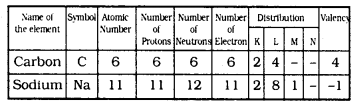Question 2.
If K and L shells of an atom are full, then what would be the total number of electrons in the atom?
2 electrons are there in K shell.
8 electrons are there in L shell.
∴ Total No. of Electrons = 2 + 8 = 10

Question 1.
How will you find the valency of chlorine, sulphur and magnesium?
i) Chlorine atom has 7 electrons in its outermost shell. Its valency is 7. But it is easier for chlorine to gain one electron instead of losing seven electrons. Hence its valency is determined by subtracting seven electrons from the octet and this gives us a valency of one for chlorine.
ii) Sulphur atom has 6 electrons in its outermost shell. But it is easier for sulphur to gain two electrons instead of losing 6 electrons. Hence its valency is determined by subtracting 6 electrons from the octet and this gives us a valency of sulphur is two.
iii) Outermost orbit of Megnesium has 2 electrons, hence it has valency two.

Question 1.
If number of electrons in an atom is 8 and number of protons is also 8, then

1. what is the atomic number of the atom
2. What is the charge on the atom?

1. Atomic number of the atom is 16.
2. Charge on the atom is zero.

Question 2.
With the help of Table 4.1, find out the mass number of oxygen and sulphur atom.
As per Table 4.1 mass number of oxygen is 16 and mass number of sulphur is 32.

Question 1.
For the symbol of H, D and T tabulate three sub-atomic particles found in each of them.
H means protium ($$_{1}^{1} \mathrm{H}$$), deuterium ($$_{1}^{2} \mathrm{H}$$ or D), tritium ($$_{1}^{3} \mathrm{H}$$ or T) the ato, In $$_{1}^{1} \mathrm{H}$$ there is only, 1 proton and 1 electron.
In Deuterium, $$_{1}^{2} \mathrm{H}$$ there is one proton, one electron and 1 neutron.

Question 2.
Write the electronic configuration of any one pair of isotopes and isobars.
Isotopes: Chlorine has two isotopes $$_{35}^{17} \mathrm{H}$$Cl and $$_{37}^{17} \mathrm{H}$$Cl.
Electronic configuration: Electronic configuaration of two chlorine atoms is as follows: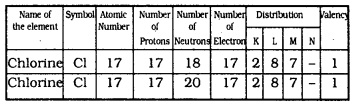ii) Isobars : Let us consider two elements – calcium atomic number 20, and argon, atomic number 18. The number of electrons in these atoms is different, but the mass number of both these elements is 40. They differ in Electronic configuration.

### KSEEB Solutions for Class 9 Science Chapter 4 Textbook Exercises

Question 1.
Compare the properties of electrons, protons, and neutrons.

 Electron Proton Neutron It has a negative charge Proton is positively charged These do not have any charge

Question 2.
What are the limitations of J.J. Thomson’s model of the atom?
Although Thomson’s model explained that atoms are electrically neutral, the results of experiments carried out by other scientists could not be explained by this model.

Question 3.
What are the limitations of Rutherford’s model of the atom?
The revolution of the electron in a circular orbit is not expected to be stable. Any particle in the circular orbit would undergo acceleration. During acceleration, charged particles would radiate energy. Thus, the revolving electron would lose energy and finally fall into the nucleus. If this were so, the atom should be highly unstable, and hence matter would not exist in the form that we know., we know that atoms are quite stable.

Question 4.
Describe Bohr’s model of the atom.
Neils Bohr put forward the following postulates about the model of an atom.

1. Only certain special orbits known as discrete orbits of electrons are allowed inside the atom.
2. While revolving in discrete orbits the electrons do not radiate energy.

Question 5.
Compare all the proposed models of an atom given in this chapter.
i) Thomson proposed that An atom consists of a positively charged sphere and the electrons are embedded in it.
ii) Rutherford put forward the nuclear model of an atom, which had the following features:
(a) There is a positively charged centre in an atom called the nucleus. Nearly all the mass of an atom resides in the nucleus.
(b) The electrons revolve around the nucleus in circular paths.
(c) The size of the nucleus is very small as compared to the size of the atom.
iii) Neils Bohr put forward the following postulates about the model of an atom.
a) Only certain special orbits known as discrete orbits of electrons are allowed inside the atom.
b) While revolving in discrete orbits the electrons do not radiate energy.
iv) In 1932, J. Chadwick discovered another sub-atomic particle which had no charge and a mass nearly equal to that of a proton. It was eventually named as a neutron. Neutrons Eire present in the nucleus of all atoms, except hydrogen. Atom has 3 subatomic particles called
(a) Protons
(b) Neutrons
(c) Electrons

Question 6.
Summarise the rules for the writing of distribution of electrons in various shells for the first eighteen elements.
The following rules are followed for writing the number of electrons in different energy levels or shells.
i) The maximum number of electrons present in a shell is given by the formula 2n2, where ‘n’ is the orbit number or energy level index, 1,2, 3… Hence the maximum number of electrons in different shells are as follows:
First orbit or K-shell will be = 2 × 12 × 2, Second orbit or L shell will be = 2 × 22 = 8, third orbit or M-shell will be = 2 × 32 = 18, fourth orbit or N-shell will be = 2 × 42 = 32 and so on.
ii) The maximum number of electrons that can be accommodated in the outermost orbit is 8.
iii) Electrons are not accommodated in a given shell unless the inner shells are filled. That is the shells are filled in a step-wise manner.

Question 7.
Define valency by taking examples of silicon and oxygen.
i) The electronic configuration of silicon is as follows: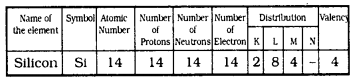Silicon has four electrons in the outermost orbit. So its valency is 4.
ii) Electronic configuration of oxygen is as follows: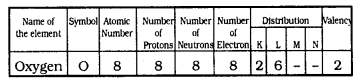Oxygen has 6 electrons in the outermost orbit, but it looses 4 electrons. Hence its valency is 2.

Question 8.
Explain with examples:
(i) Atomic number
(ii) Mass Number,
(iii) Isotopes and
(iv) Isobars. Give any two uses of isotopes.
(i) Atomic Number: It is the number of protons of an atom. This is denoted by ‘Z’. For example, Hydrogen has 1 proton and its atomic number is 1.
(ii) Mass Number: Protons and neutrons are present in the nucleus of an atom. These are also called nucleons. Therefore the sum of protons and neutrons in the nucleus is called Mass Number. For example, the mass of carbon is 12u because it has 6 protons and 6 neutrons, 6u + 6u = 12u.
(iii) Isotopes: Atoms of the same element having the same atomic number but different mass numbers are called isotopes.
Eg: There are three isotopes of hydrogen atom namely protium, deuterium, and tritium.
(iv) Isobars: Atoms of different elements with different atomic numbers, which have the same mass number are known as isobars.
Eg: Atomic number of calcium is 20, and Argon, has atomic number 18. The number of electrons in these atoms is different, but the mass number of both these elements is 40. That is the total number of nucleons is the same in the atoms of this pair of elements.
Uses of isotopes are

1. An isotope of uranium is used as a fuel in nuclear reactors,
2. An isotope of cobalt is used in the treatment of cancer.

Question 9.
Na+ has completely filled K and L shells. Explain.
Electronic configuration of sodium: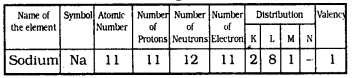Sodium has 2 electrons in K shell and 8 electrons in L shell. Hence both orbits are completely filled.

Question 10.
If bromine atom is available in isotopes $$_{79}^{35} \mathrm{H}$$ (49.7%) and $$_{81}^{35} \mathrm{H}$$Br (50.3%) calculate the average atomic mass of bromine atom.
As per data, average atomic mass of bromine is
(79 × 49.7% + 81 × 50.3%)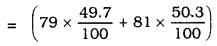= 392.63 + 407.43
= 800.06
Average of this means $$\frac{800.06}{2}$$ = 400.03.
The average atomic mass of bromine atom is 400.03.

Question 11.
The average atomic mass of a sample of an element X is 16.2u. What are the percentage of isotopes $$_{16}^{8} \mathrm{H}$$ and $$_{18}^{8} \mathrm{H}$$ in the sample?
The average atomic mass of a sample X= 16.2 u.
Let the % of isotope of $$_{16}^{8} \mathrm{H}$$ be y and % of isotope of y = (100 – y)%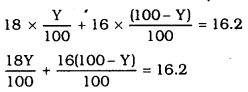fi 18y + 1600 = 16y = 1620
fi 2y = 1620 – 1600
∴ 2y = 20
y = 10
Percentage of isotope X = 10%
Percentage of isotope y = 100- 10 = 90%.

Question 12.
If Z = 3, what would be the valency of the element? Also name the element.
Z = 3 means its valency is 1. That element is Lithium.

Question 13.
Composition of the nuclei of two atomic species X and Y are given as under.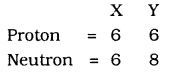Give the mass numbers of X and Y. What is the relation between the two species?
Mass Number of X species = 6u + 6u = 12u
Mass Number of Y species = 6u + 8u = 14u
Both spcies has equal number of protons but they differ in neutrons.

Question 14.
For the following statements, write T for True and F for False.
a) J.J. Thomson proposed that the nucleus of an atom contains only nucleons.
b) A neutron is formed by an electron and a proton combining together. Therefore it is neutral.
c) The mass of an electron is about 1 /2000 times that of proton.
d) An isotope of Iodine is used for making tincture iodine, which is used as a medicine.
a) True.
b) True.
c) False
d) True.

Question 15.
Rutherford’s alpha-particle scattering experiment was responsible for the discovery of
a) Atomic Nucleus
b) Electron
c) Proton
d) Neutron
a) Atomic Nucleus.

Question 16.
Isotopes of an element have
a) the same physical properties.
b) different chemical properties.
c) different number of neutrons
d) different atomic numbers
c) different number of neutrons.

Question 17.
Number of valance electrons in Cl ion are
a) 16
b) 8
c) 17
d) 18
a) 17.

Question 18.
Which one of the following is a correct electronic configuration of sodium?
a) 2, 8
b) 8, 2, 1
c) 2, 1, 8
d) 2, 8, 1
a) 2, 8

Question 19.
Complete the following table: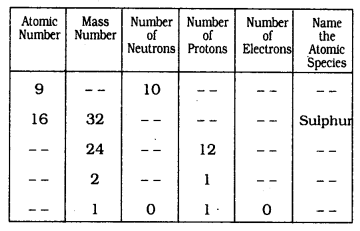### KSEEB Solutions for Class 9 Science Chapter 4 Additional Questions

Question 1.
Draw a neat diagram of Thomson’s Atomic model and label the parts.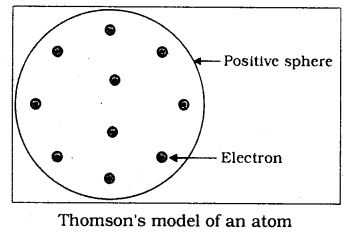Question 2.
Draw a neat diagram of energy levels in a atom and lable the parts.Question 3.
Name the scientist who discovered Neutrons.